# Wipro Elite NTH Coding Questions | PrepInsta

## Wipro NTH Coding Questions and Answer

Wipro Elite NTH Coding Questions and Answer are not like a general Programming Round Questions with Solutions it is all together different from C programming.

We have analyzed over 100+ Wipro NTH Coding Questions. Below you will find Similar pattern based Wipro NTH Coding Round Questions, Wipro NTH Coding Questions that are asked constantly in Wipro NTH Placement test. The languages that you can use in the test are C , C++ , Java and Python.Total number of questions 2 questions Total Time Duration 60 minutes Type of Test Adaptive Negative Marking No

### WIPRO NTH PROGRAMMING MODEL QUESTIONS AND ANSWERS

1. Wipro NTH C Programming Questions for On Campus
2. Wipro NTH Java Programming Questions for On Campus
3. Wipro NTH C++ Programming Questions for On Campus

### Free Material

• Program to calculate the GCD of two numbers –
• Program to calculate the GCD of three numbers –
• Program to check if the given number is Prime or not –
• Program to Display the distinct elements of an array –
• Program to Sort first half in ascending order and second half in descending order in an array –
• Program for Matrix multiplication  –
• Program to Remove vowels from an input string –
• Program to Print Sum of all Odd Numbers present in a larger number –
• Program to Print the Transpose of a matrix –
• Program to Display the largest element in each row in a 2-D Array –
• Program to Check the balance of Parenthesis –

### Related Banners

Get PrepInsta Prime & get Access to all 200+ courses offered by PrepInsta in One Subscription

## Some Coding Questions for Practice

### Question 1

Wipro’s client eBay wants to run a campaign on their website, which will have the following parameters, eBay wants that on certain x products, they want to calculate the final price, for each product on eBay there will be a stock unit parameter, this parameter will denote, how many items are their in their fulfillment center

Now, while these numbers if are positive means product x is available in the fulfillment center and if not than the product is not available and cannot be shipped to the customer

Now the price on for each product varies based on the distance of the customer from the fulfillment center. Now, each product is in different fulfillment zone. Now, these values are 00’s kms for each centurion km. The price available would further increase by factor distance.

You’ve to find the maximum discount price for each product if the product can be shipped.

Following are the input/output parameters :

Input

•  The first line of the input will contain number of products.
• The second line will contain price for each of these products.
• The third line contains shipping distance in 00’s kms
• The fourth line contains SKU’s

Output

• It will contain the final price for each deliverable item in SKU’s

Example :

Input:

• 6
• 87 103 229 41 8 86
• 3 1 9 2 1 2
• 7 -21 30 0 -4 -3

Output

• 261 2061

### Question : 2

(asked in Wipro On Campus, Sastra University, Aug, 2019)

Problem: in this first line you are required to take the value of m and n as the number of rows and columns of matrix, then you are required to take the input elements of array.
As an output you are required to print the sum of each row then the row having the maximum sum.
Test Case :
Input : 3 3
1 2 3
4 5 6
7 8 9
Output :
Row 1 : 6
Row 2 : 15
Row 3 : 24
Row 3 is having the maximum sum : 24

### Question : 3

(asked in Wipro On Campus, PESIT Bangalore, Oct, 2019)

Problem: You are required to count the number of words in a sentence.
You are required to pass all the test cases

Test Cases :
Test Case : 1
Input : welcome to the world
Output : 4

Test Case : 2
Input : [space] say hello
Output : 2

Test Case : 3
Input : To get pass you need to study hard [space] [space]
Output : 8

### Question : 4

(asked in Wipro On Campus, VIT University, Aug, 2019)

Problem: First of all you need to input a whole sentence in the string, then you need to enter the target
word.
As an output you need to find the number of times that particular target word is repeated in the
sentence.

Test Cases :

Test Case : 1
Input : welcome world to the new world
world
Output : 2

Test Case : 2
Input : working hard and working smart both are different ways of working
working
Output : 3

### Question : 5

(asked in Wipro On Campus, MIET Meerut, Aug, 2019)

Problem Statement

You are required to implement the following function:
Int SumNumberDivisible(int m, int n);

The function accepts 2 positive integer ‘m’ and ‘n’ as its arguments.
You are required to calculate the sum of numbers divisible both by 3 and 5, between ‘m’ and ‘n’ both inclusive and return the same.

Note
0 < m <= n

Example
Input:
m : 12
n : 50
Output   90

Explanation:
The numbers divisible by both 3 and 5, between 12 and 50 both inclusive are
{15, 30, 45} and their sum is 90.

Sample Input

m : 100
n : 160

Sample Output

510

### Question : 6

(asked in Wipro On Campus, Galgotia University Noida, Aug, 2019)

Input
The first line of input consists of three space separated integers. Num, start and end representing the size of list [N], the starting value of the range and the ending value of the range respectively.
The second line of input consists N space separated integers representing the distances of the employee from the company.

Output
Print space separated integers representing the ID’s of the employee whose distance liew within the given range else return -1.

Example
Input
6 30 50
29 38 12 48 39 55

Output
1 3 4

Explanation :
There are 3 employees with id 1, 3, 4 whose distance from the office lies within the given range.

### Question : 7

Write a code for the following functions
Function 1 : sortArray
It’s function is to sort the passed array with a particular length, there are 2 parameters passed into it first is the length of array and next is the elements of list.
Function 2 : findMaxElement
It’s function is to get the 2 array with their own particular elements and length, and find the maximum element from both the array and print it.

Input
12
2 5 1 3 9 8 4 6 5 2 3 11
11
11 13 2 4 15 17 67 44 2 100 23

Output
100

### Question 8

(asked in Wipro On Campus, HBTI Kanpur, Sep, 2019)

Problem: you are given a number, and you have to extract the key by finding the difference between the sum of the even and odd numbers of the input.

Test Case :

Input : 24319587

Output :  11

Explanation : odd terms : 3 + 1 + 9 + 5 + 7 = 25

even terms : 2 + 4 + 8 = 14

output : 11 (25-14)

### Question 9

Problem:  in this first line you are required to take the value of m and n as the number of rows and columns of matrix, then you are required to take the input elements of array.

As an output you are required to print the sum of each row then the row having the maximum sum.

Test Case :

Input : 3 3

1 2 3

4 5 6

7 8 9

Output :

Row 1 : 6
Row 2 : 15
Row 3 : 24

Row 3 is having the maximum sum : 24

### Question 10

Problem:  in this first line you are required to take the input that set the number of elements to be inserted, the next line takes the elements as input.

You are required to print the sum of maximum and minimum element of the list

Test Case :

Input : 6

55 87 46 21 34 79

Output :

108

Explanation :

21 + 87 = 108

### Question 11

Problem:  in this first line you are required to take the input of an integer number, and in the second line you are required to input the target element

You are required to print the number of time target element occured in the integer

Test Case :

Input : 734139

3

Output :

2

Explanation :

3 occured 2 times in the integer.

## Paid Material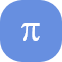Wipro NTH Coding  Question 1Wipro NTH Coding  Question 2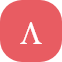Wipro NTH Coding  Question 3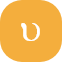Wipro NTH Coding Question 4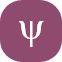Wipro NTH Coding Question 5Wipro NTH Coding Question 6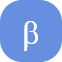Wipro NTH Coding Question 7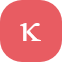Wipro NTH Coding Question 8Wipro NTH Coding Question 9Wipro NTH Coding Question 10Wipro NTH Coding Question 11Wipro NTH Coding Question 12Wipro NTH Coding Question 13Wipro NTH Coding Question 14Wipro NTH Coding Question 15Wipro NTH Coding Question 16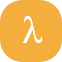Wipro NTH Coding Question 17Wipro NTH Coding Question 18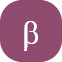Wipro NTH Coding Question 19Wipro NTH Coding Question 20Wipro NTH Coding Question 21Wipro NTH Coding Question 22Wipro NTH Coding Question 23Wipro NTH Coding Question 24Wipro NTH Coding  Question 25Wipro NTH Coding  Question 26Wipro NTH Coding  Question 27Wipro NTH Coding Question 28Wipro NTH Coding  Question 29Wipro NTH Coding  Question 30Wipro NTH Coding  Question 31Wipro NTH Coding Question 32Wipro NTH Coding Question 33Wipro NTH Coding Question 34Wipro NTH Coding Question 35Wipro NTH Coding Question 36Wipro NTH Coding Question 37Wipro NTH Coding Question 38Wipro NTH Coding Question 39Wipro NTH Coding Question 40

## Get over 200+ course One Subscription

Courses like AI/ML, Cloud Computing, Ethical Hacking, C, C++, Java, Python, DSA (All Languages), Competitive Coding (All Languages), TCS, Infosys, Wipro, Amazon, DBMS, SQL and others

## Checkout list of all the video courses in PrepInsta Prime Subscription

### 127 comments on “Wipro Elite NTH Coding Questions | PrepInsta”

•rohitvatson632002

python code for 4th question:
sentence=list(input().strip().split(” “))
sword=input()
c=0
for i in sentence:
if sword==i:
c+=1
print(c)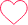0
•4028-

question number 9 in python
n,m=map(int,input().split())
sum1=0
count=0
a=[]
for i in range(n):
b=[]
for j in range(1):
b=list(map(int,input().split()))
a.append(b)
for i in range(n):
sum1=0
for j in range(m):
sum1+=a[i][j]
count+=1
print(“Row{} {}”.format(count,sum1))0